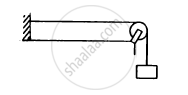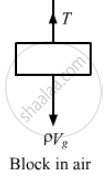Department of Pre-University Education, KarnatakaPUC Karnataka Science Class 11
Advertisement Remove all ads

# Following Figure Shows a String Stretched by a Block Going Over a Pulley. the String Vibrates in Its Tenth Harmonic in Unison with a Particular Tuning For. When a - Physics

Sum

Following figure shows a string stretched by a block going over a pulley. The string vibrates in its tenth harmonic in unison with a particular tuning for. When a beaker containing water is brought under the block so that the block is completely dipped into the beaker, the string vibrates in its eleventh harmonic. Find the density of the material of the block.Advertisement Remove all ads

#### Solution

Density of the block = ρ
Volume of block = V
∴ Weight of the block is, W = ρVg
∴ Tension in the string, T = W

The tuning fork resonates with different frequencies in the two cases.
Let the tenth harmonic be f10.$f_{11} = \frac{11}{2L}\sqrt{\frac{T'}{m}}$
$= \frac{11}{2L}\sqrt{\frac{\left( \rho - \rho_w \right) Vg}{m}}$
The frequency (f) of the tuning fork is same.

$\therefore f_{10} = f_{11}$

$\Rightarrow \frac{10}{2L}\sqrt{\frac{\rho Vg}{m}} = \frac{11}{2L}\sqrt{\frac{\left( \rho - \rho_\omega \right) Vg}{m}}$

$\Rightarrow \frac{100}{121} = \frac{\rho - 1}{\rho} \left( because \rho_\omega = 1 gm/cc \right)$

$\Rightarrow 100 \rho = 121 \rho - 121$

$\Rightarrow \rho = \frac{121}{21} = 5 . 8 gm/cc$

$= 5 . 8 \times {10}^3 kg/ m^3$
Therefore, the required density is $5 . 8 \times {10}^3 kg/ m^3$

Is there an error in this question or solution?
Advertisement Remove all ads

#### APPEARS IN

HC Verma Class 11, 12 Concepts of Physics 1
Chapter 15 Wave Motion and Waves on a String
Q 55 | Page 327
Advertisement Remove all ads
Advertisement Remove all ads
Share
Notifications

View all notifications

Forgot password?